# 非完整约束Hamilton动力系统保结构算法*

(大连理工大学 工程力学系; 工业装备结构分析国家重点实验室, 辽宁 大连 116023)

## 1 非完整约束系统及Lagrange-d’Alembert原理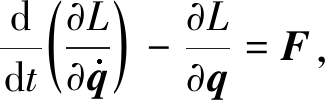(1)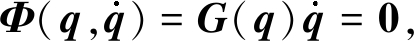(2)

F=GT(q)λ,

(3)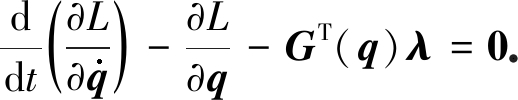(4)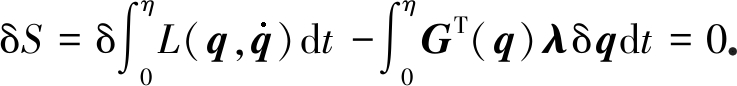(5)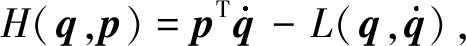(6)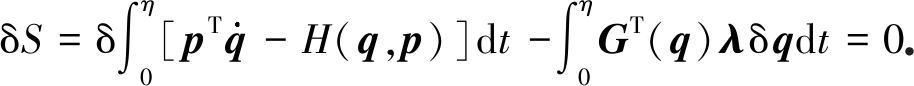(7)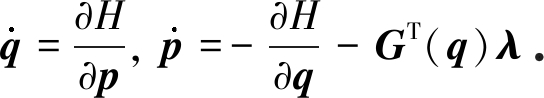(8)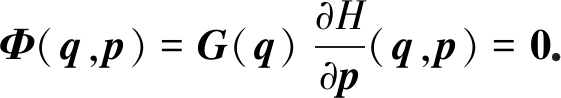(9)

## 2 非完整约束系统保结构算法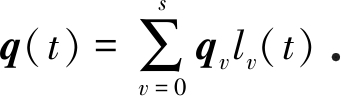(10)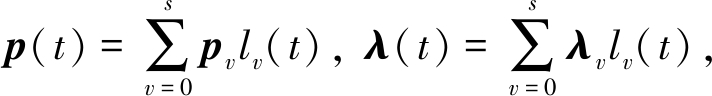(11)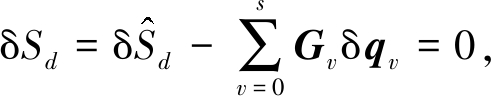(12)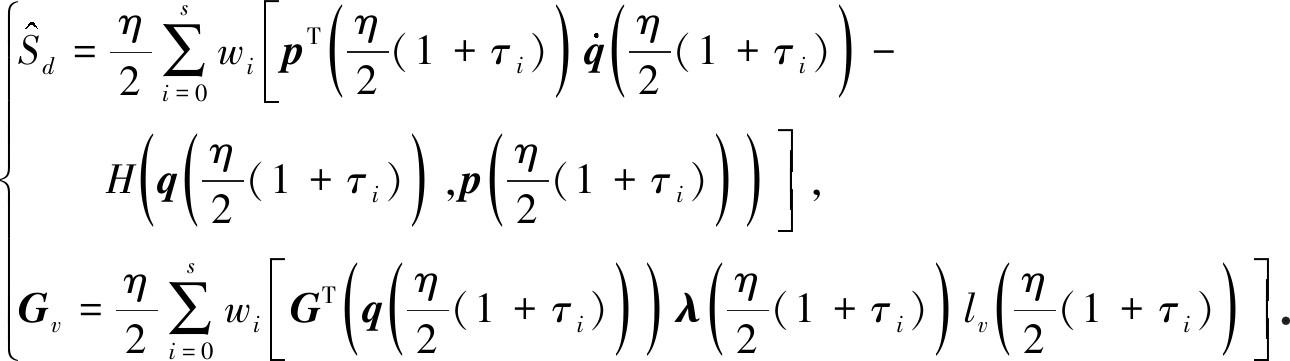(13)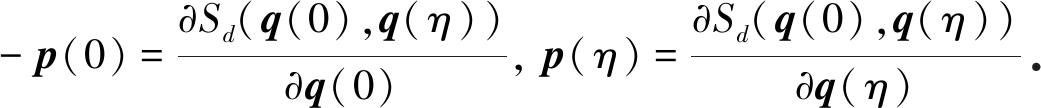(14)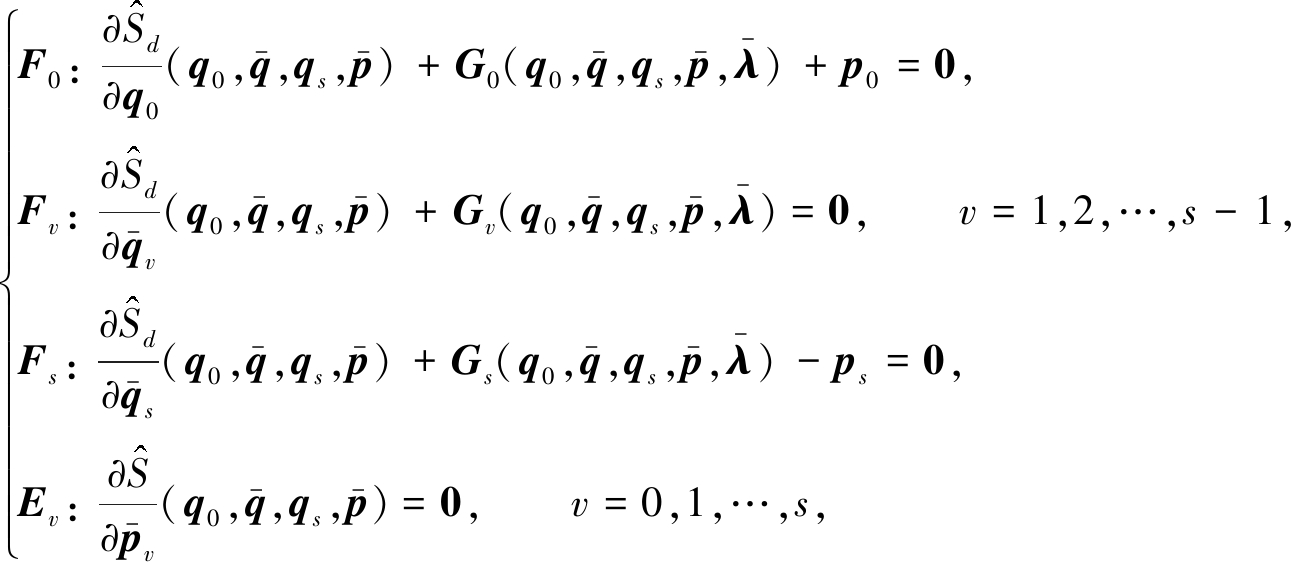(15)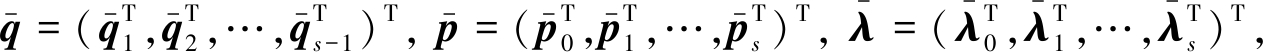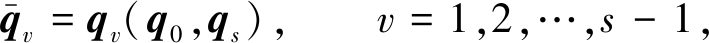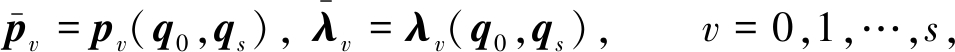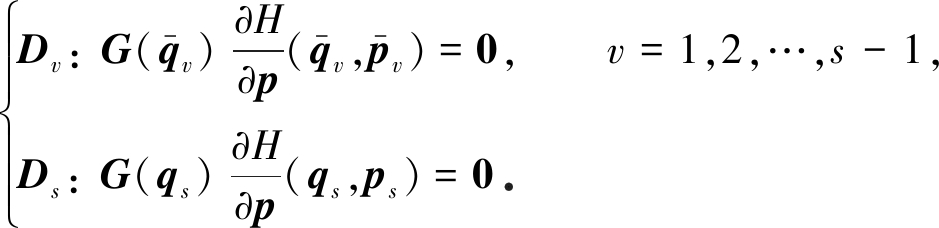(16)

## 3 对称性证明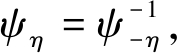(17)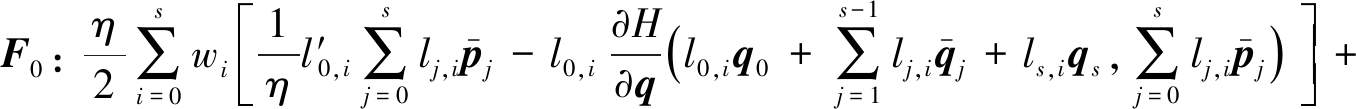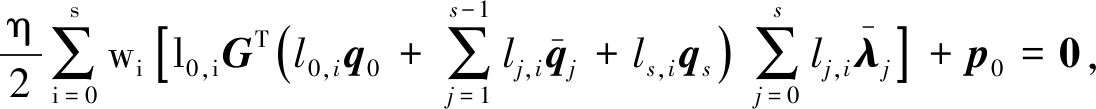(18)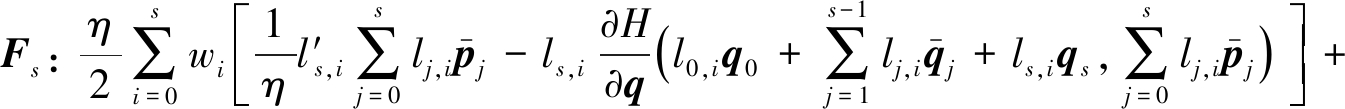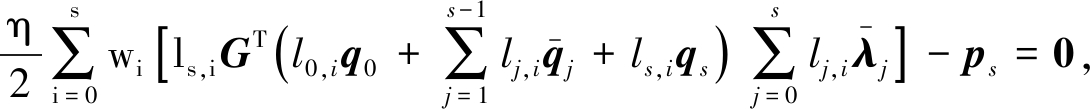(19)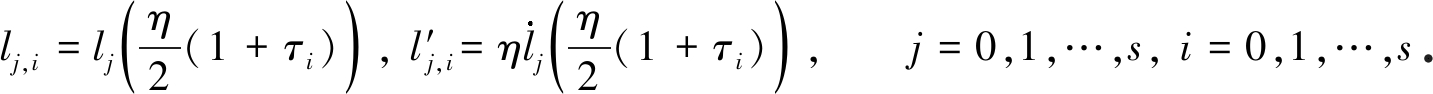(20)

lj,i=ls-j,s-i, lj,i=-ls-j,s-i, τi=-τs-i, wi=ws-i

(21)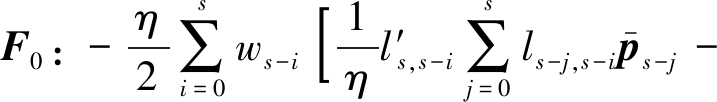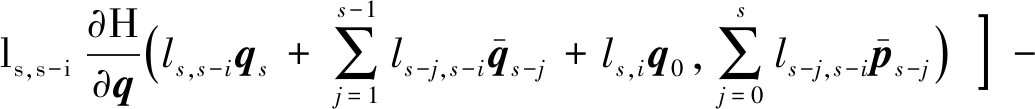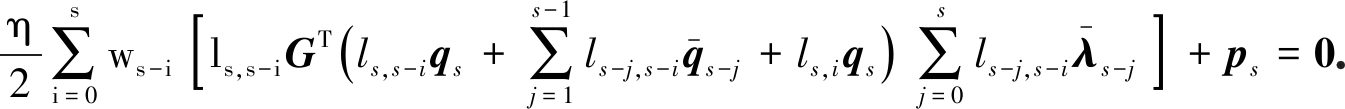(22)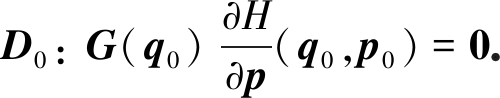(23)

## 4 数 值 算 例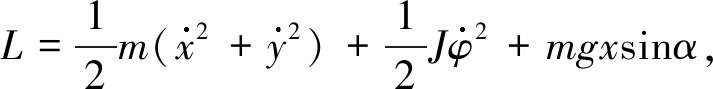(24)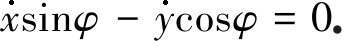(25)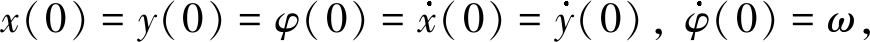(26)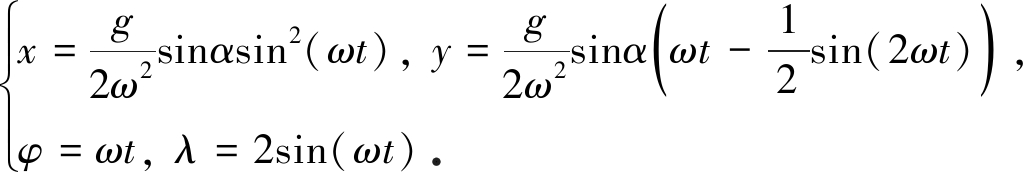(27)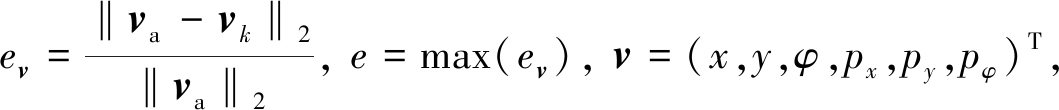(28)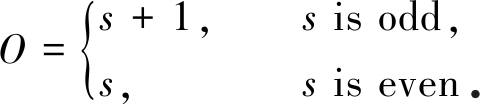(29)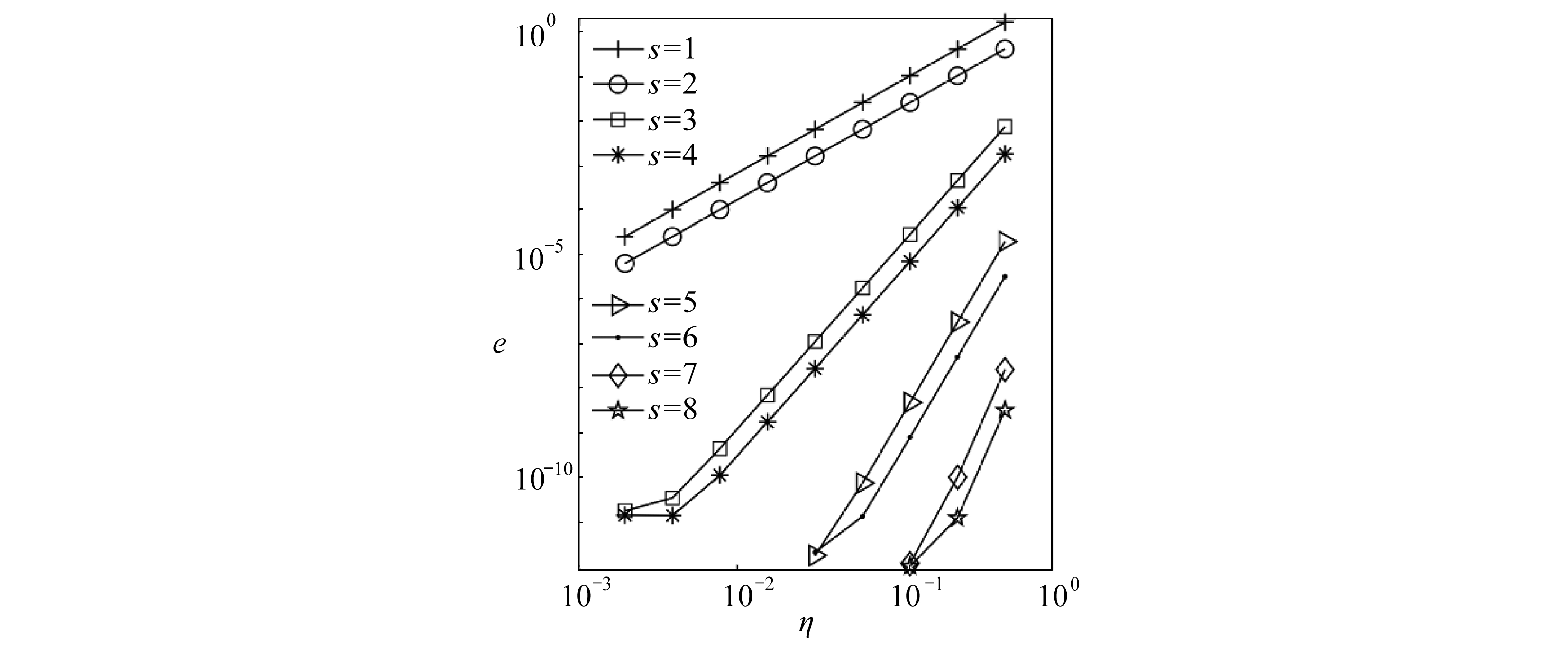Fig. 1 The global errors of state vectors with different orders of interpolation s and step sizes η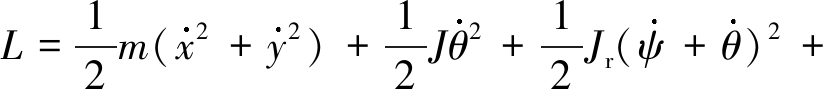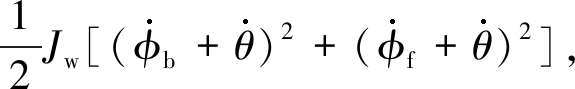(30)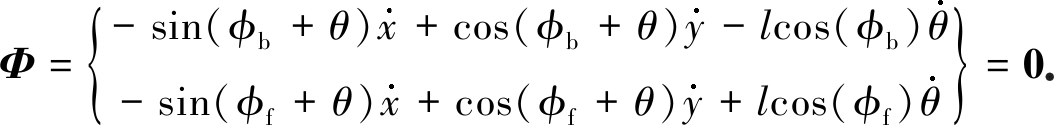(31)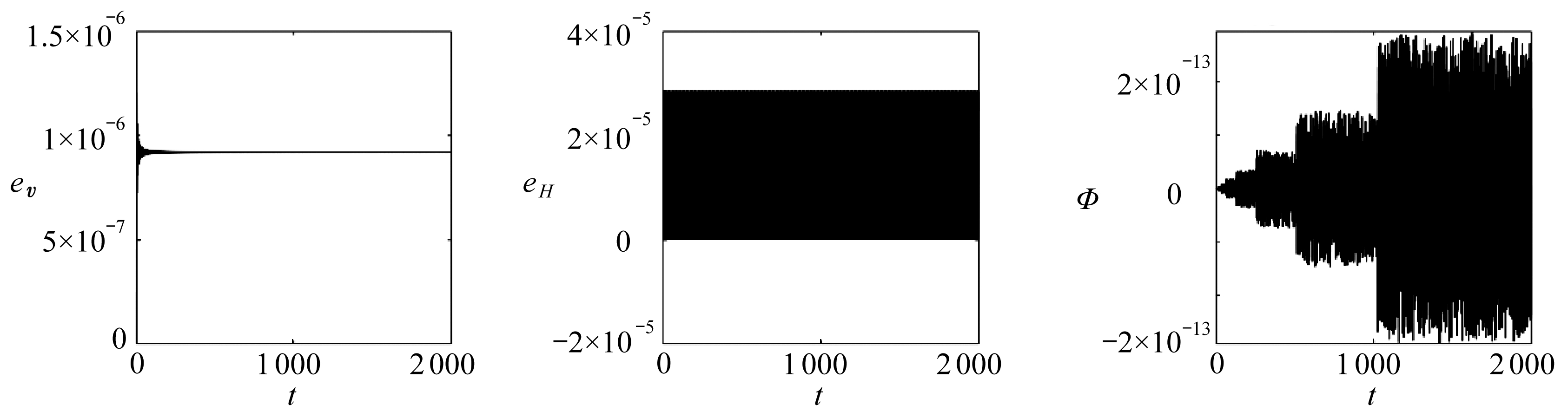(a) 状态向量相对误差 (b) Hamilton函数相对误差 (c) 非完整约束
(a) The relative error of the state vector (b) The relative error of the Hamiltonian function (c) The nonholonomic the state vector constraint

Fig. 2 Numerical results of the skate for long-time simulation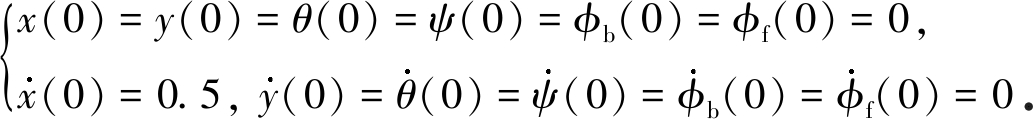(32)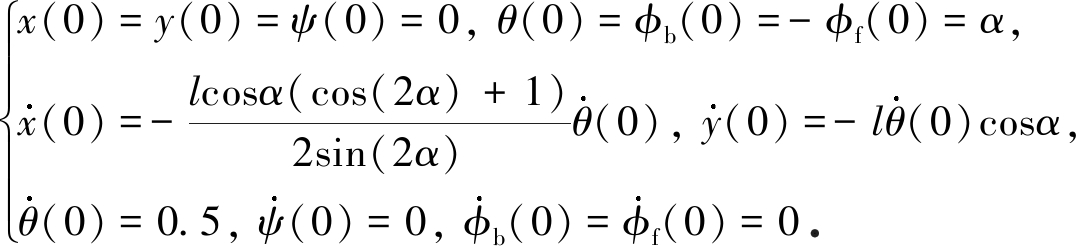(33)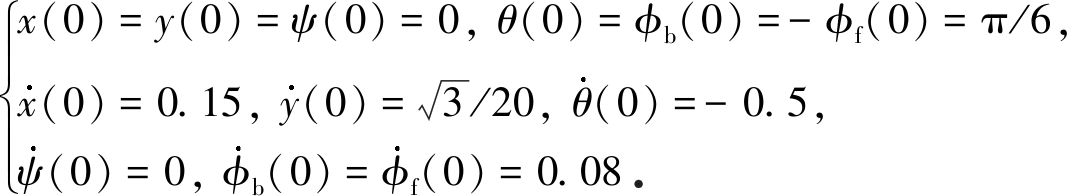(34)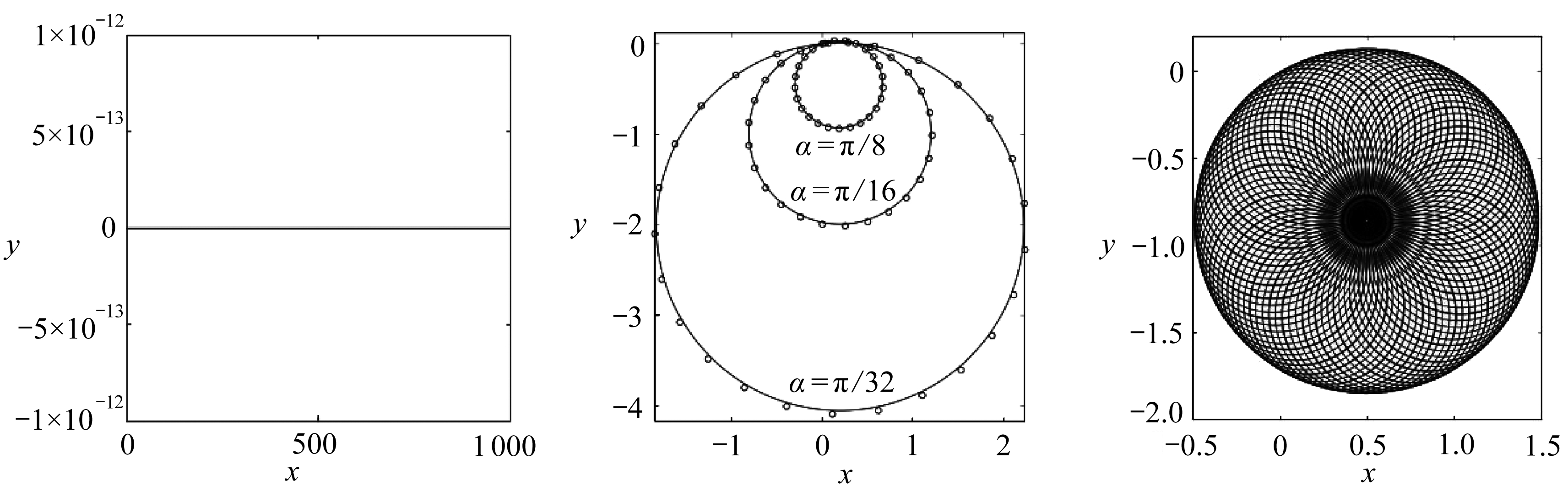(a) 第一种初值条件(b) 第二种初值条件 (c) 第三种初值条件
(a) The 1st initial condition (b) The 2nd initial condition (c) The 3rd initial condition

Fig. 3 The orbits of the snakeboard(a) α=π/8 (b) α=π/16 (c) α=π/32

Fig. 4 The nonholonomic constraints of the snakeboard for s=1,η=1/2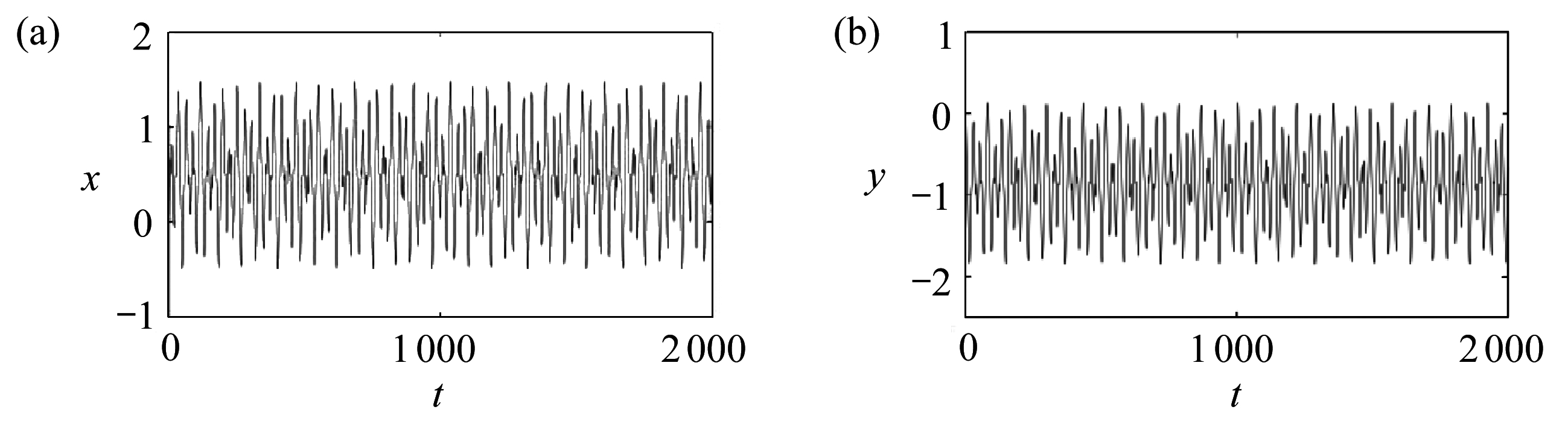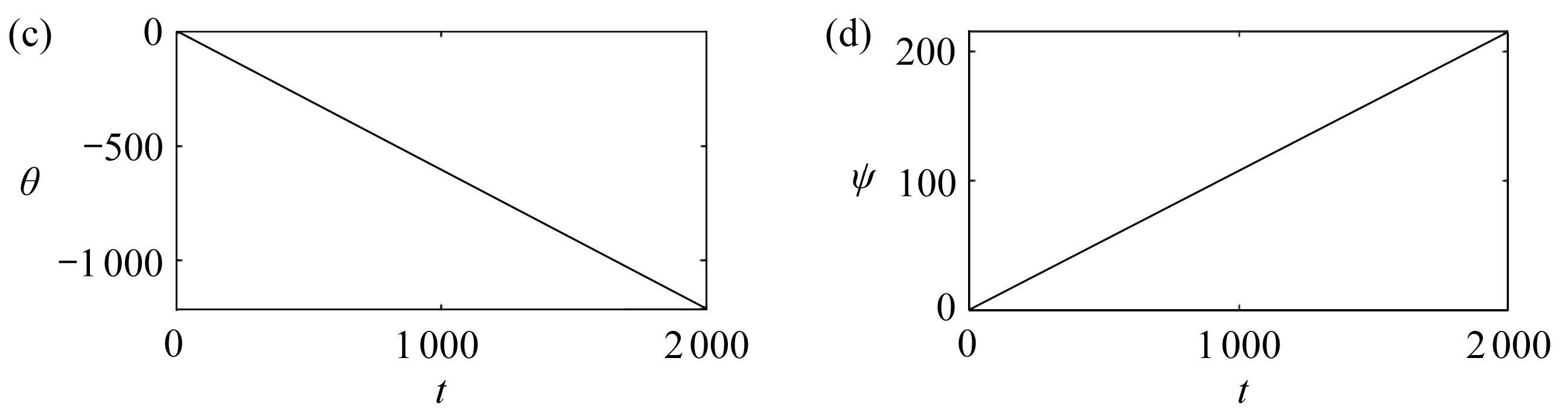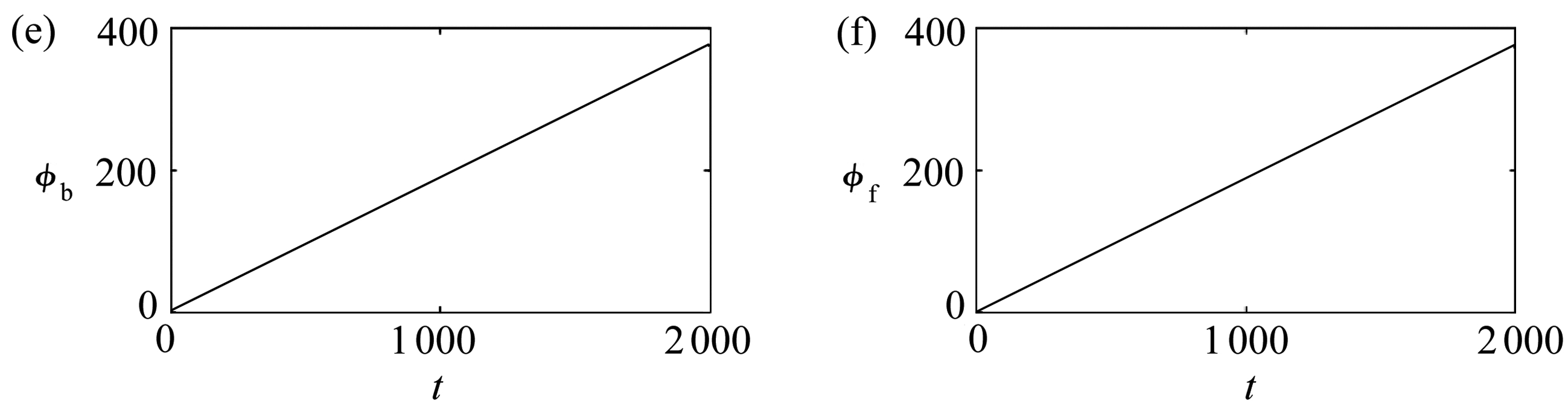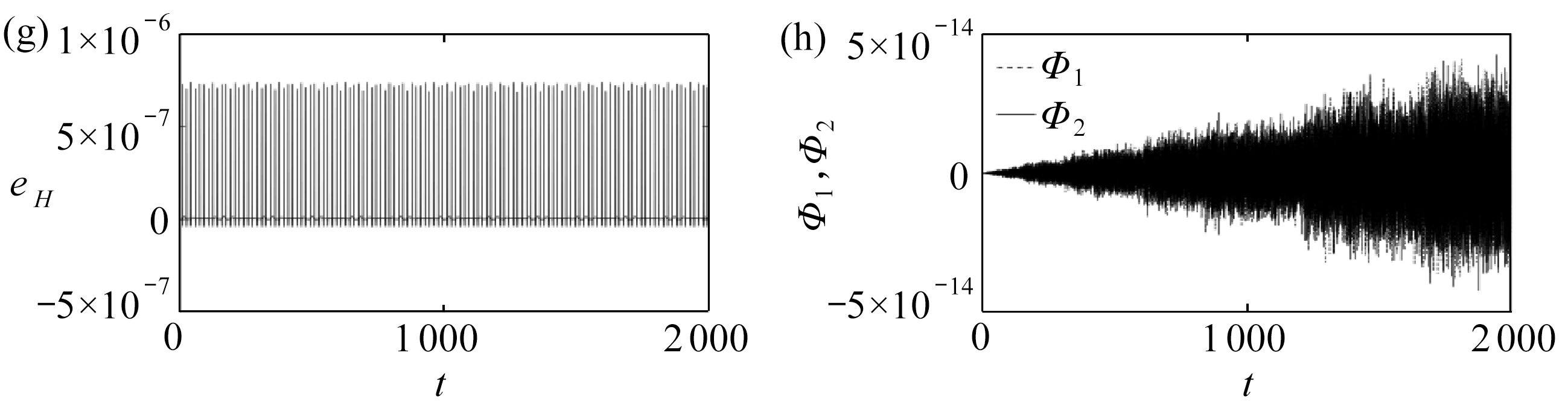Fig. 5 Numerical results of the snakeboard for long-time simulation with the 3rd initial condition

## 5 结 论

 HERTZ A, GARBASSO A. Die prinzipien der mechanik in neuem zusammenhang dargestellt[J]. Il Nuovo Cimento (18951900), 1895, 1(1): 40-59.

 APPELL P. Traité de Mécanique Rationnelle[M]. Gauthier-Villars, 1924.

 HAMEL G. Theoretische Mechanik[M]. Berlin: Springer, 1978.

 BLOCH A M. Nonholonomic Mechanics and Control[M]. Berlin: Springer, 2015.

 MARSDEN J E, WEST M. Discrete mechanics and variational integrators[J]. Acta Numerica, 2001, 10(1): 357-514.

 LEW A, MARSDEN J E, ORTIZ M, et al. Variational time integrators[J]. International Journal for Numerical Methods in Engineering, 2010, 60(1): 153-212.

 HAIRER E, WANNER G, LUBICH C. Geometric Numerical Integration[M]. Berlin: Springer, 2002.

 CORTÉS J, MART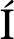NEZ S. Non-holonomic integrators[J]. Nonlinearity, 2001, 14(5): 1365-1392.

 CORTÉS J. Energy conserving nonholonomic integrators[J]. Discrete & Continuous Dynamical Systems, 2003, 2003(S): 189-199.

 DE LE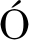N M, DE DIEGO D M, SANTAMARIA-MERINO A. Geometric integrators and nonholonomic mechanics[J]. Journal of Mathematical Physics, 2002, 45(3): 1042.

 DE LEN M, DE DIEGO D M, SANTAMARIA-MERINO A. Geometric numerical integration of nonholonomic systems and optimal control problems[J]. European Journal of Control, 2004, 10(5): 515-521.

 MCLACHLAN R, PERLMUTTER M. Integrators for nonholonomic mechanical systems[J]. Journal of Nonlinear Science, 2006, 16(4): 283-328.

 KOBILAROV M, MARSDEN J E, SUKHATME G S. Geometric discretization of nonholonomic systems with symmetries[J]. Discrete and Continuous Dynamical Systems(Series S), 2010, 3(1): 61-84.

 GAO Q, TAN S J, ZHANG H W, et al. Symplectic algorithms based on the principle of least action and generating functions[J]. International Journal for Numerical Methods in Engineering, 2012, 89(4): 438-508.

 HALL J, LEOK M. Spectral variational integrators[J]. Numerische Mathematik, 2012, 130(4): 681-740.

 HAIRER E, LUBICH C, WANNER G. Geometric Numerical Integration: Structure-Preserving Algorithms for Ordinary Differential Equations[M]. Berlin: Springer, 2006.

 OSTROWSKI J, LEWIS A, MURRAY R, et al. Nonholonomic mechanics and locomotion: the snakeboard example[C]//Paper Presented at the Proceedings of the 1994 IEEE International Conference on Robotics and Automation. Piscataway, USA, 1994.

# A Structure-Preserving Algorithm for Hamiltonian Systems With Nonholonomic Constraints

MAN Shumin, GAO Qiang, ZHONG Wanxie

(State Key Laboratory of Structural Analysis for Industrial Equipment; Department of Engineering Mechanics, Dalian University of Technology, Dalian, Liaoning 116023, P.R.China)

Abstract: Based on the concept of variational integrator and the Lagrange-d’Alembert principle with dual variables, a high-order structure-preserving algorithm for Hamiltonian systems with nonholonomic constraints was proposed. Based on the variational integrator, a discretization form of the Lagrange-d’Alembert principle with dual variables was obtained by means of appropriate polynomials and quadrature rules. On the basis of this discretization form, a numerical integration method was given with displacements at both ends of the integral interval as independent variables. Meanwhile, the nonholonomic constraints were strictly met at the endpoints of the integral interval and the control points within the interval. The symmetric property of the proposed algorithm was proved. Numerical examples show that, the proposed algorithm has a high convergence order, strictly meets the nonholonomic constraints and has good long-time behaviors.

Key words: nonholonomic constraint; variational integrator; structure-preserving algorithm; dual variables

*收稿日期： 2019-12-23

(我刊编委钟万勰来稿)

DOI: 10.21656/1000-0887.400375

(Contributed by ZHONG Wanxie, M. AMM Editorial Board)

Foundation item: The National Natural Science Foundation of China(11972107;91748203)